# Basic Probability

in STEMGeeks8 months ago

Hello there. In this probability post, I go over some basics of probability. The reader should be comfortable with fractions at the minimum.

Reference: Data Management 12 by Wayne Erdman, Maria Rosa Cruiscuolo, Roland Meisel, David Petro, Jacob Speijer, Wendy Telford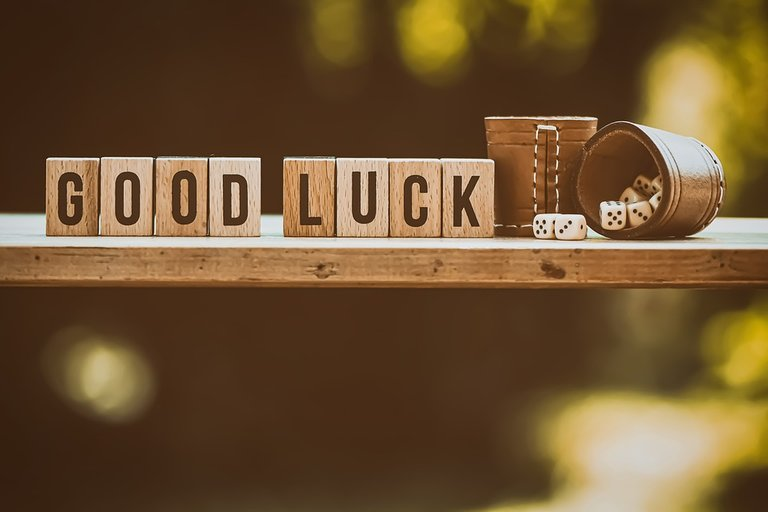Pixabay Image Source

## Contents

• What Is Probability?
• Basic Examples
• Complements And The Keyword "Not"
• Practice Problems

## What Is Probability?

The field of probability deals with measuring or trying to measure the likelihood of unknown events occurring.
Probabilities can be based on theory (theoretical), observations from experiments (experimental) or even from beliefs/guessing (subjective).

Experimental probabilities focus on the probability of a desired outcome occurring. This probability is expressed as a fraction where the numerator is the number of desired outcomes that has occurred from an experiment. The denominator for this probability represents the number of trials in the experiment.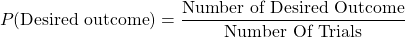A subjective probability is based on belief, intuition and maybe even some guessing in the mix. These probabilities generally do not involve much math nor data. Examples of subjective probabilities include:

• I an 100% sure I got an A on that test.
• There is no way (0%) that she cheated on him.
• I think this MMA fight is 50-50.

Some subjective probabilities may have better predictions where data analysis, statistics, mathematics are behind the predicted probabilities. These more informed probabilities occur in sports odds, MMA fighting odds, politics, weather forecasting, financial markets forecasting.

This post will look more at theoretical probabilities (classical probabilities). These probabilities are based on analyzing on possible outcomes for an event. A coin has two outcomes being heads or tails when flipped. A regular six-sided die has the numbered outcomes 1, 2, 3, 4, 5 or 6 and would land on one of them when rolled.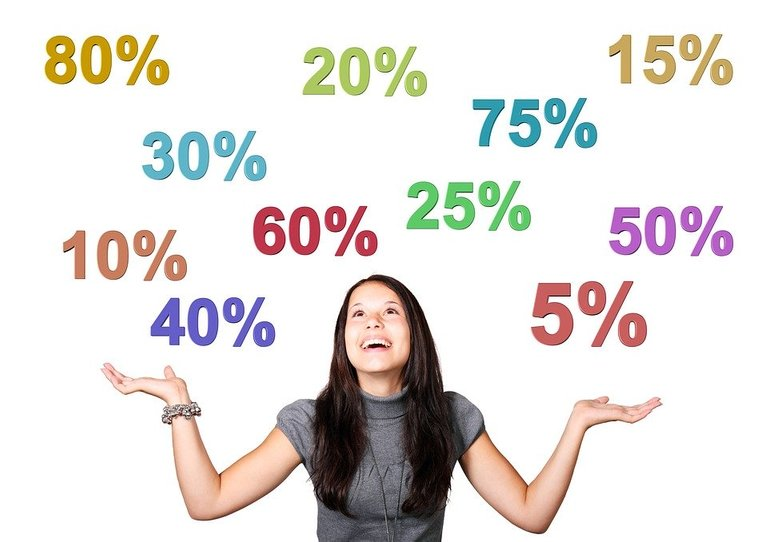Pixabay Image Source

## Basic Examples

Example One

The results from a fair spinner with four colours are displayed in a table. There were 50 spins.

ColourCountPercentage
Red1224%
Blue1530%
Green714%
Black1632%

The percentage column displays the experimental probabilities for each of the four colours. As an example, twenty-four percent for red was obtained from the fraction 12/50 for 12 landings of red out of 50 spins.

Example Two

The probability of landing heads on a fair coin is one half or 50%. For tails, the probability is 50% as well. These are theoretical probabilities. There are two outcomes for the flipped coin and once a coin is flipped it can only land on one of two coins.

Example Three - Picking A Marble From A Bag

There are ten marbles in a bag. Out of the ten marbles in the bag, there are 4 red marbles, 5 green marbles and one yellow marble. Markus randomly selects a marble from the bag (without looking).

• The probability of randomly picking a red marble is 4 out of 10 or 40%.
• The probability of randomly picking a green marble is 5 out of 10 or 50%.
• Ten percent is the probability of picking a yellow marble.
• It is impossible (0% probability) of choosing a blue marble.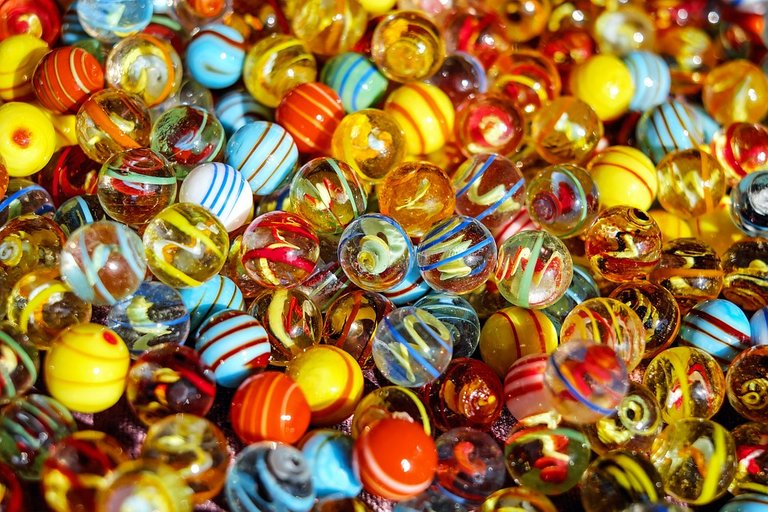Pixabay Image Source

## Complements And The Keyword "Not"

In probability, the concept of a complement is based on the keyword not. The keyword not means pretty much the same thing as in English. Not a horse means anything that is different from a horse.

A key equation to remember for complements is that the probability of event A occurring plus the probability of event A not occurring is equal to 1.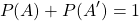where A represents the event that occurs and A' represents the event that does not occur.

You can rearrange the above formula to obtain these two variations: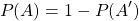or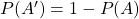Example One

The probability of rolling not a 6 on a six sided die is 5 out of 6. This is from one minus the probability of rolling a six.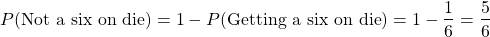Example Two

Consider the word `PINEAPPLE`. You have SCRABBLE nine letter tiles that form the word `PINEAPPLE`. The probability of randomly selecting a letter tile that is not the letter P is 1 minus 3/9. This computes to the answer of 6/9 or two-thirds.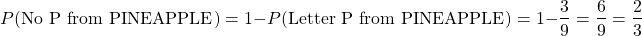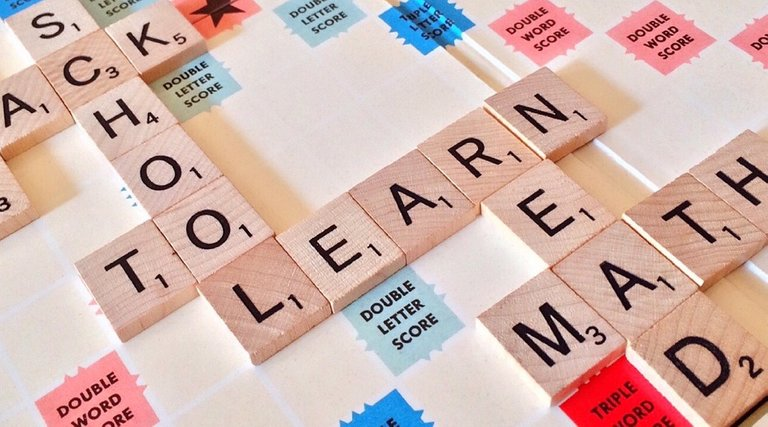Pixabay Image Source

## Practice Problems

1. On a six sided die, what is the probability of rolling an even number?

2. On a six sided die, what is the probability of rolling a number greater than 2?

3. There are seven pairs of socks in Susie's drawer. Two pairs are pink, three are purple and the remaining two pairs are white. Susie picks a pair at random. What is the probability of the randomly selected pairs of socks are pink?

4. Refer to the Susie scenario in problem three. What is the probability of the randomly selected pairs of socks are not white?

5. You have a twelve sided die. What is the probability of rolling a number that is not a factor of 8?

## Solutions To Practice Problems

1. 1/2

2. 4/6 or 2/3

3. 2/7

4. 5/7

5. The factors of 8 are 1, 2, 4 and 8. This makes 4 numbers that you do not want. The probability of rolling a number that is not a factor of 8 from a 12 sided die is 8 out of 12 or two-thirds.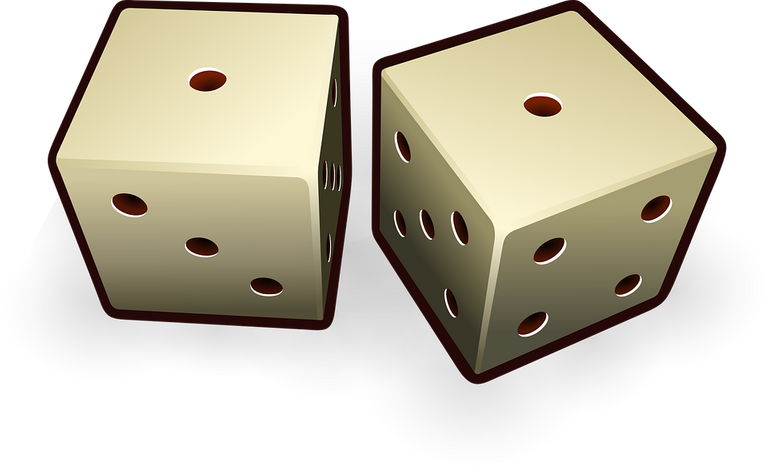Pixabay Image Source